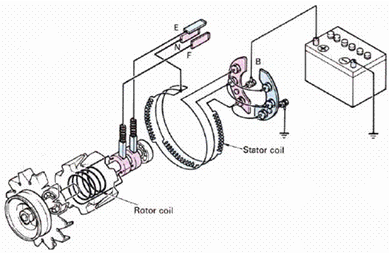Home > English > Basic Principles of Alternator Work

# Basic Principles of Alternator Work

Figure 1.13 shows how the AC voltage is generated by electromagnetic induction. In this picture the magnetic field rotates and the conductor is formed into a coil. When the conductor is in a parallel position with a magnetic field, the conductor is not cut off by any magnetic field, at this rotation does not produce current and voltage at all. In Figure 1.13 B, we see the magnetic field has been rotated 90 º, placing it on the right side of the conductor.Figure 1.13 AC current generated from rotation of magnetic field

At this point, we see the maximum number of flux lines cut off the conductor at the north pole, inducing the voltage at the maximum positive value. When the magnetic field is turned 90 degrees apart, the conductor returns parallel to the magnetic field (Fig. 1.13 C). Again the voltage drops to zero because there is no flux line cut off the conductor.

90º Other rotations of the magnetic field position the magnetic field on the top conductor (Fig. 1.13 D). At this point in rotation, it is the line of the south pole flux that cuts the conductor, producing a voltage at the maximum negative value. The advanced rotation of the other 90º brings to the end of one complete rotation. At the end of this rotation, the zero voltage again as the conductor is parallel to the magnetic field. Referring back to Figure 1.13 we can see the voltage waves from time to time by placing the waves at 360º rotation of the magnetic field (rotor).

Very little voltage and current are produced with a rotating magnet inside a single wire / wire node. If the knot of wire / magnet and magnet are placed inside the iron frame, then a channeling line for the magnetic force line is created. Since iron supplies magnetism very easily, adding iron skeletons will greatly increase the number of line forces between the poles of N and the pole S (see Figure 1.14).Figure 1.14 Cage rotor

A large number of magnetic force lines are at the center of the magnetic end. Therefore, the strong magnetic field is at the center of the magnet and the weak magnetic field is on the front and rear edge. This condition will occur when the air gap between the magnet and the framework of the plane is larger at the front and rear edge than in the center of the magnet The amount of voltage induced within the conductor is proportional to the number of force lines that cut through the conductor in a given time period. Voltage / voltage will also increase if the magnetic rod rotates faster, because the line force cuts through the wire / wire in a shorter period of time.Figure 1. 15 The rotating magnet inside the alternator is called the rotor and the wire / wire node and the assembly frame is called the stator (Fig. 1.15)."Tasikmalaya, Indonesia"